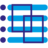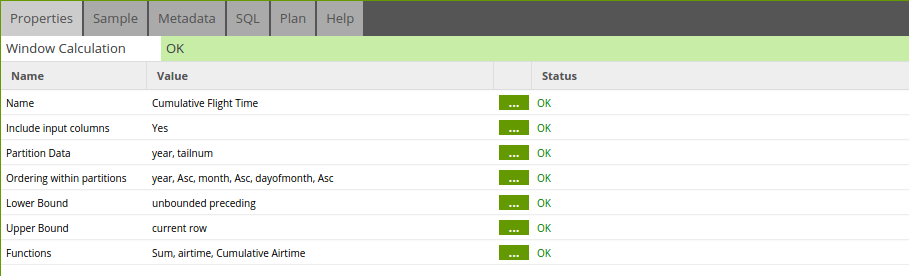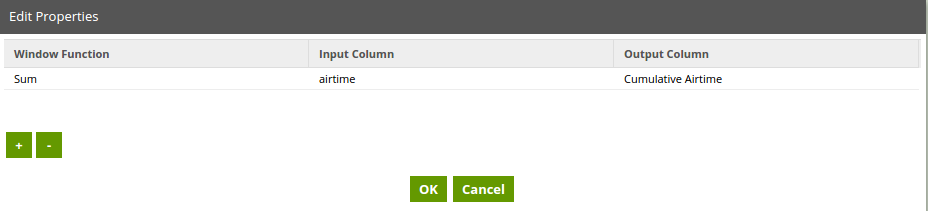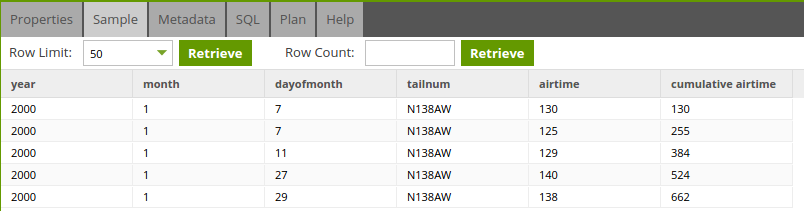• 1.46
Window Calculation Component

# Window Calculation Component## Window Calculation Component

Allows the user to set up a calculation using a window function. Window functions allow users to query tables for a partition of a data set, termed a 'window'.

## Properties

Property Setting Description
Name Text The descriptive name for the component.
Include Input Columns Yes/No Defines whether the component passes all input columns into the output.
Partition Data List of Columns Defines how the input data is partitioned in order to perform the window calculation. The calculation is then performed on each partition.
Ordering within partitions Input Column The input column name for sorting within the partitioned data. Note: you can drag to reorder.
Ordering The order of the sorting: Ascending (Asc) or Descending (Desc).
You can also choose how to sort any null values with the Nulls First and Nulls Last parameters.
Lower Bound Multi Select List unbounded preceding - (default) indicates that the window starts at the first row of the partition
current row - indicates the window starts at the current row.
offset preceding - indicates that the window starts a number of rows (offset) before the current row.
Upper Bound Multi Select List unbounded following - indicates that the window ends at the last row of the partition.
current row - indicates the window starts at the current row.
offset following - indicates that the window ends a number of rows (offset) after the current row.
Functions Window Function A window function to apply (see supported functions above).
Input Column The input column that the window function will be applied to.
Output Column The name of the output column that the window function will create.

## Strategy

Generates a select statement with a window function in line using the OVER keyword. More details...

Generates a select statement with a window function in line using the OVER keyword. More details...

Generates a select statement with a window function in line using the OVER keyword. More details...

## Supported Functions

Window functions provide application developers the ability to create analytic business queries more efficiently. Window functions operate on a partition or "window" of a data set, and return a value for every row in that window.

Matillion ETL currently supports the following window functions:

• Average - returns the average (arithmetic mean) of the input column values in the window. More...
• Count - returns a count of the non-null values for the specified field. More...
• First Value - Given an ordered set of rows, returns the specified column value with respect to the first row in the window frame. More...
• Last Value - Given an ordered set of rows, returns the specified column value with respect to the first row in the window frame. More...
• Minimum - returns the minimum of the input expression values. The MIN function works with numeric values and ignores NULL values. More...
• Maximum - returns the maximum of the input expression values. The MAX function works with numeric values and ignores NULL values. More...
• Sum - returns the sum of the input column in the window. More...
• Sample Variance - return the sample variance of a set of numeric columns. More...
• Population Variance - return the sample variance of a set of numeric columns. More...
• Standard Deviation- return the standard deviation of a set of numeric values. More...
• Population Standard Deviation - return the population standard deviation of a set of numeric values. More...
• Median - calculate the median value for the range of values in a window or partition. Null values in the range are ignored. More...
• Median - calculate the median value for the range of values in a window or partition. Null values in the range are ignored. More...

The following functions are supported by the Rank Component.

• Rank - determines the rank of a value in a group of values.
• Dense Rank - determines the rank of a value in a group of values. The Dense Rank function differs from rank in one respect: If two or more rows tie, there is no gap in the sequence of ranked values.
• Cumulative Distribution - determines the cumulative distribution of a value within a window or partition.
• Percent Rank - Calculates the percent rank of a given row.
• Row Number - Determines the ordinal number of the current row within a group of rows, counting from 1.

## Example

This example uses the flight data to add a new column with the cumulative flight time (airtime).

Note: The filter just shows one plane for clarity however this can be removed and the function will remain accurate for the whole dataset.The component properties are set up as below. The data is partitioned by year then tailnum and ordered the same way (the ordering doesn't really add anything in this scenario).

The lower bound of unbounded preceding and the upper bound of current row define a window that starts from the current year and tailnum up to the current row hence a cumulation for a particular plane in a particular year.The function used is a sum that acts on the airtime input column and outputs a new column Cumulative Airtime.The Sample data shows the new column added.